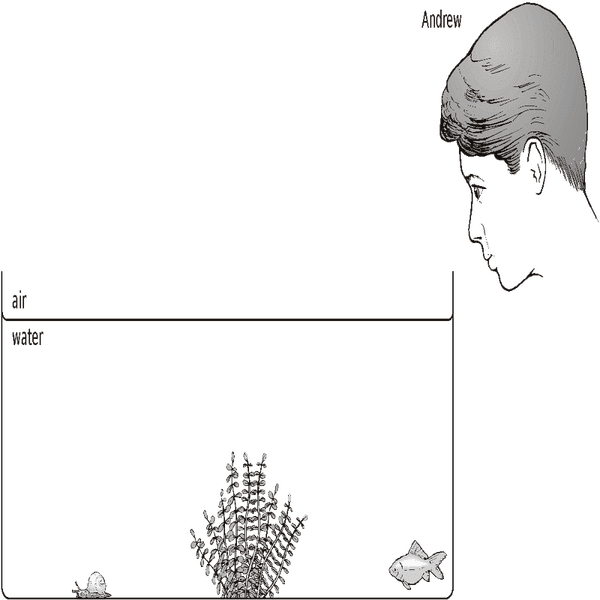# Angle of refraction

IBNU1

## Homework StatementI need to find how a ray of light will travel from the snail towards Andrews eye.
I need to find the angle of incidence and angle of refraction.
I know the index of refraction of both materials
Air: 1
Water 1.33

## Homework Equations

Snells law
1.33 *sin(X) = sin(y)
x is angle of incidence
y is angle of refraction

## The Attempt at a Solution

I only know how to solve these problems when I have a normal line
How do I find the normal line[/B]

Staff Emeritus
Homework Helper
Gold Member

## Homework Statement

I need to find how a ray of light will travel from the snail towards Andrews eye.
I need to find the angle of incidence and angle of refraction.
I know the index of refraction of both materials
Air: 1
Water 1.33

## Homework Equations

Snells law
1.33 *sin(X) = sin(y)
x is angle of incidence
y is angle of refraction

## The Attempt at a Solution

I only know how to solve these problems when I have a normal line
How do I find the normal line
Hello IBNU1. Welcome to PF .

Do you have any dimensions for this ?

•IBNU1
IBNU1
Hello IBNU1. Welcome to PF .

Do you have any dimensions for this ?
Sorry I forgot to include them
The depth of the water is 5m and the distance from his eye to the snail is 25 m

Staff Emeritus